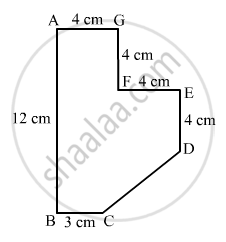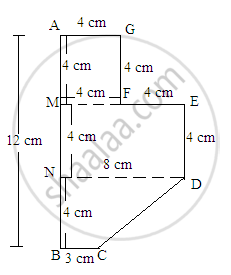Share

# Find the Area of the Field Shown in Fig. 20.39 by Dividing It into a Square, a Rectangle and a Trapezium. - Mathematics

Course
ConceptArea of Trapezium

#### Question

Find the area of the field shown in Fig. 20.39 by dividing it into a square, a rectangle and a trapezium.#### Solution

The given figure can be divided into a square, a parallelogram and a trapezium as shown in following figure:From the above figure:

Area of the figure=(Area of square AGFM with sides 4 cm)+(Area of rectangle MEDN with length 8 cm and width 4 cm)+(Area of trapezium NDCB with parallel sides 8 cm and 3 cm and perpendicular height 4 cm

$= (4\times4)+(8\times4)+[\frac{1}{2}\times(8+3)\times(4)]$

$= 16+32+22$

${= 70 cm}^2$

Is there an error in this question or solution?

#### APPEARS IN

RD Sharma Solution for Mathematics for Class 8 by R D Sharma (2019-2020 Session) (2017 to Current)
Chapter 20: Mensuration - I (Area of a Trapezium and a Polygon)
Ex. 20.2 | Q: 20 | Page no. 24

#### Video TutorialsVIEW ALL 

Solution Find the Area of the Field Shown in Fig. 20.39 by Dividing It into a Square, a Rectangle and a Trapezium. Concept: Area of Trapezium.
S